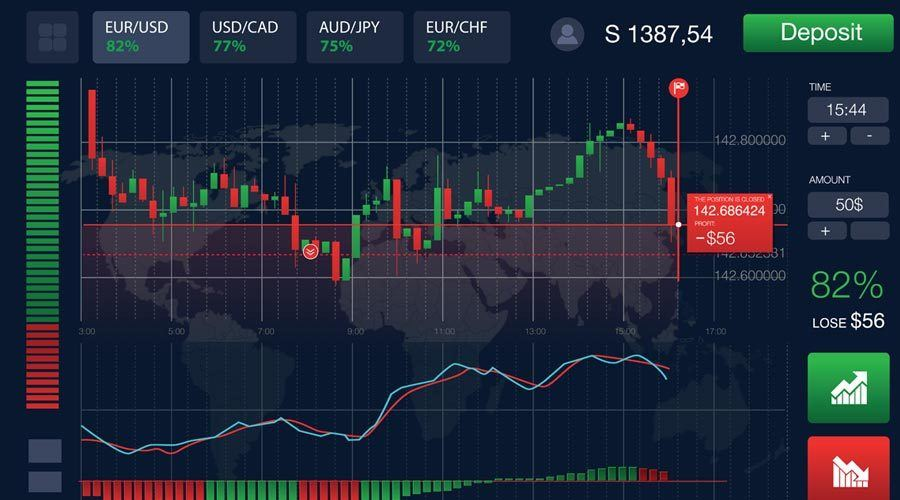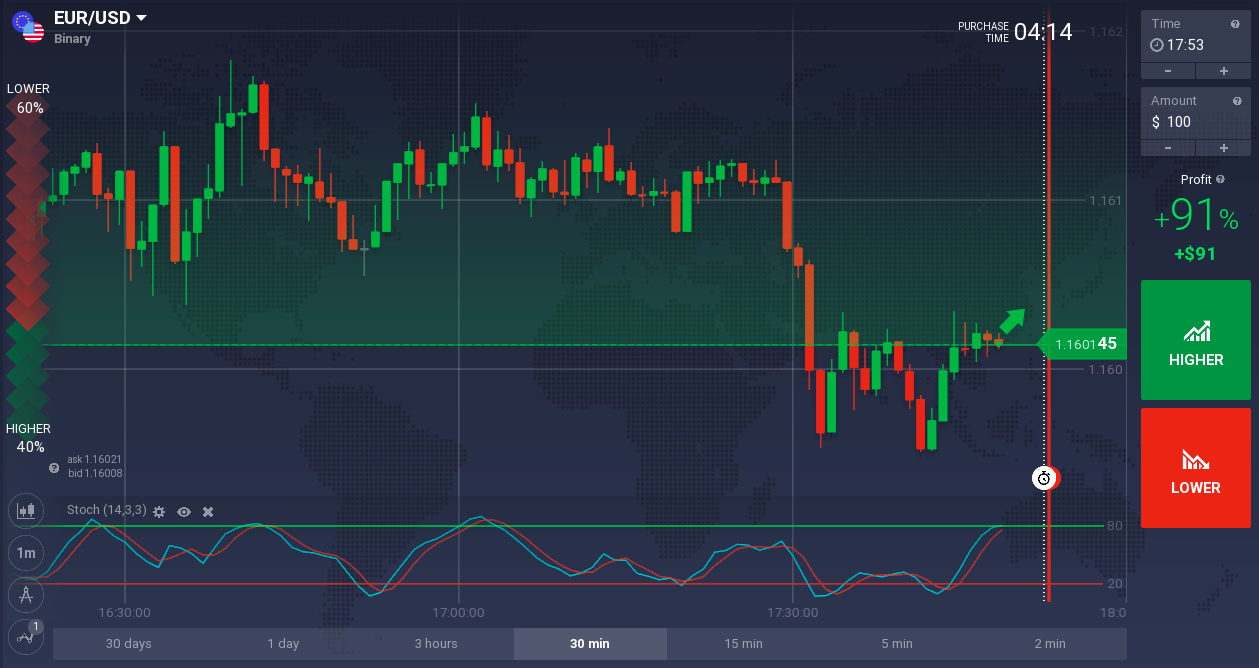# Strategy Correlations between assets for binary options

The strategy of correlation between assets is a very different strategy than the usual binary options strategies. In fact, usually binary options strategies require the use of one or more technical analysis tools, such as indicators or oscillators. But in this case, the asset correlation strategy requires a different approach. In fact, when we talk about correlation, we mean the link that usually exists between two different assets. But what do we mean by “linking assets” and how can they be two assets, even different categories (such as a currency pair and a raw material), connected to each other?

First of all, when we talk about a link between assets, we refer to the price movements of the two assets. This strategy in fact exploits these links, that is, the correlations between the movement of the value of an asset and the movement of the value of another asset. Put simply, there is a correlation when for example the price of an asset increases by a certain value and the price of another asset increases well or badly of the same value, all during the same period of time.

But a correlation can also be of the opposite type, that is, a price of an asset that increases and a price of another asset that falls, always of the same quantity and during the same period of time. When we talk about correlation, it means that there is a link between changes in the value of an asset and those in another asset. This reveals an important trading opportunity: in fact, if we know that if an asset X increases by a Tot of value then even a Y asset will increase by Tot value (or it will drop by Tot value), we can exploit this opportunity and invest accordingly on both assets.

But let’s proceed calmly, starting to clarify the concept of correlation between assets with some examples, to then see how to exploit the correlation in our strategy for binary options.## Asset correlation strategy: Example of correlation between assets

As we said, we have a correlation between two assets when the changes in the value of these assets are similar during a certain period of time. There are two types of correlations between assets:

• Positive correlation between assets: In this case the value of an asset follows the value of another asset. That is, if the value of the asset X increases, then the value of the asset Y also increases. And if the value of the asset X falls, then the value of the asset Y also falls. In this case the changes in value take place in the same direction. Note how the value of oil and that of Eni equities is positively correlated: the price of the two assets moves in the same direction.
• Correlation between negative assets: In this case, the value of an asset follows the value of another asset in opposite ways. That is, if the value of the asset X increases, the value of the Y asset decreases. And if the value of the asset X falls, the value of the asset Y increases. Therefore in this case the value changes take place in the opposite direction. Notice how in this case the currency pair EUR / USD and USD / CHF have a negative correlation, i.e. when the value of one increases, the value of the other decreases and vice versa.

Obviously, not all assets are related to each other. Many assets have no correlation with other assets: these assets are useless for the purposes of this binary options strategy. As you have certainly understood, the strategy of correlations between assets exploits these very bonds, which allow us to find excellent investment opportunities. In fact, if two assets are correlated, and therefore we know how they react to each other, we can then invest in both assets accordingly.For example, here are some assets that are related to each other …

• Correlation between USD and gold: Here we have a negative correlation, because gold is traded in USD dollars. Therefore, when the dollar falls, the price of gold rises. While if the value of the dollar increases, then the price of gold falls.
• Correlation between Australian AUD dollar and gold: In this case we have a positive correlation, given that Australia is also an important gold exporter. For this reason, many traders choose to trade with the AUD / USD currency pair according to the following reasoning: if the value of gold increases, then traders invest upwards on the AUD / USD pair; vice versa, if the value of gold decreases, then they invest down on the AUD / USD pair.
• Correlation between Canadian CAD and oil: Here we have a positive correlation, because the Canadian economy is strongly linked to oil, given that Canada has large reserves of oil and is one of the largest oil producers in the world. Therefore, if the price of oil increases, the value of the Canadian dollar also increases; if instead the price of oil decreases, then the value of the Canadian dollar decreases.
• Correlation between USD and oil: In this case we have a negative correlation, because oil is traded in US dollars and the United States is also one of the biggest oil importers. This means that if the value of the US dollar increases, then the value of oil drops; vice versa, if the US dollar falls, the value of oil increases. In this case, traders often also use the USD / CAD currency pair to take advantage of this correlation: if the price of oil tends to rise, then traders will invest downward on the USD / CAD pair, and in the opposite case, invest upwards on the USD / CAD pair when the oil price drops.

## Asset correlation strategy conclusions for binary options

This strategy for binary options is then used with two different assets, not with just one asset. And it is mainly based on knowing how to find two assets that have a correlation between them. To find a correlation between two assets, you just use a good charting system to have financial charts at your disposal: in this way, you can view the graphs of two asset pairs and look for any correlations, both positive and negative.

Obviously, correlations do not always work. That is, even if two assets are correlated with each other it is not certain that the two assets always follow this correlation. Much also depends on the macroeconomic data released during the week, which can affect the movements of the financial markets. Therefore it is always necessary to choose carefully the correlations to follow, and to check how much these correlations are reliable in the long run. Maybe choosing a regulated binary options broker with which you can use a demo account, and test the correlations without risking your capital.Author: Chowdhury Shahid Uz Zaman
Chowdhury Shahid Uz Zaman Russell is a blogger who loves to explore new things. His passion for helping people in all aspects of daily things flows through in the respected industries coverage he provides.  He graduated from Open University with major marketing. He writes for several sites online such as Ezinearticles.com, Semrush.com, Speakymagazine.comTrendsmagazine.net. Chowdhury Shahid Uz Zaman Russell is the founder of CYONWO.com  and  Domaindoom.com.